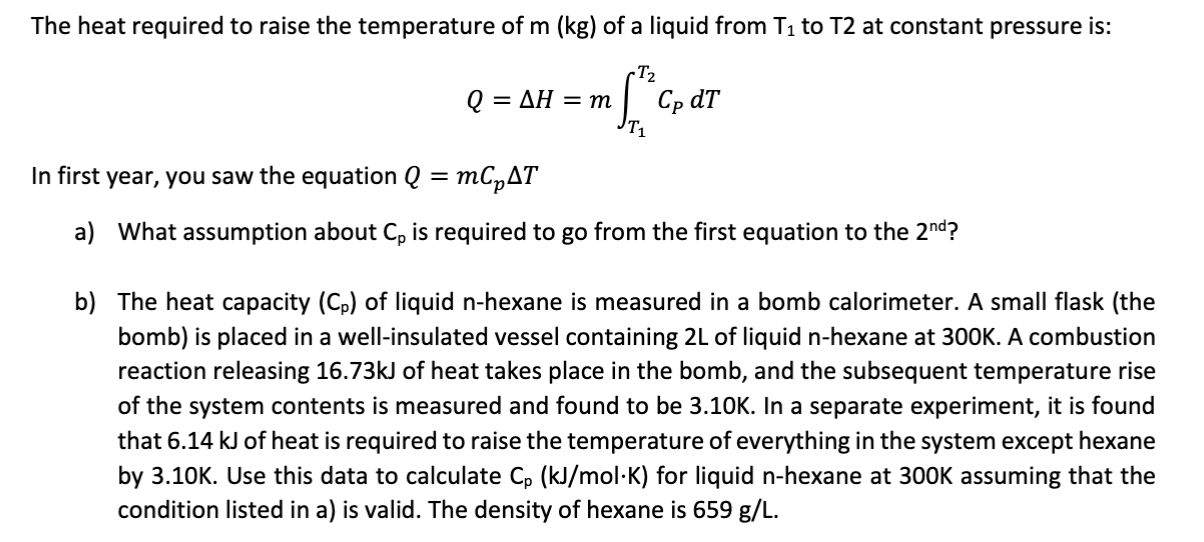# The heat required to raise the temperature of m (kg) of a liquid from T1 to T2 at constant pressure is:-T2Cp dTAH = mQ =In first year, you saw the equation Q = mCpATWhat assumption about Cp is required to go from the first equation to the 2nd?a)b) The heat capacity (Cp) of liquid n-hexane is measured in a bomb calorimeter. A small flask (thebomb) is placed in a well-insulated vessel containing 2L of liquid n-hexane at 300K. A combustionreaction releasing 16.73KJ of heat takes place in the bomb, and the subsequent temperature riseof the system contents is measured and found to be 3.10K. In a separate experiment, it is foundthat 6.14 kJ of heat is required to raise the temperature of everything in the system except hexaneby 3.10K. Use this data to calculate Cp (kJ/mol-K) for liquid n-hexane at 300K assuming that thecondition listed in a) is valid. The density of hexane is 659 g/L.

Questionhelp_outlineImage TranscriptioncloseThe heat required to raise the temperature of m (kg) of a liquid from T1 to T2 at constant pressure is: -T2 Cp dT AH = m Q = In first year, you saw the equation Q = mCpAT What assumption about Cp is required to go from the first equation to the 2nd? a) b) The heat capacity (Cp) of liquid n-hexane is measured in a bomb calorimeter. A small flask (the bomb) is placed in a well-insulated vessel containing 2L of liquid n-hexane at 300K. A combustion reaction releasing 16.73KJ of heat takes place in the bomb, and the subsequent temperature rise of the system contents is measured and found to be 3.10K. In a separate experiment, it is found that 6.14 kJ of heat is required to raise the temperature of everything in the system except hexane by 3.10K. Use this data to calculate Cp (kJ/mol-K) for liquid n-hexane at 300K assuming that the condition listed in a) is valid. The density of hexane is 659 g/L. fullscreen
check_circleExpert Solution
Step 1

There are two following equation given for calculating the heat required to raise the temperature from T1 to T2 of mass (m) of substance.

Step 2

(a) The following assumption required to go from equation (1) to (2):

When CP is assumed to be independent of temperature then term CP comes out of the integration and then expression of (2) equation is obtained.

Step 3

(b) Volume of hexane in bomb calorimeter is 2L at temperature 300 K

Case 1: System consist of liquid hexane and remaining system

∆T = 3.10 K

∆H1...

### Want to see the full answer?

See Solution

#### Want to see this answer and more?

Solutions are written by subject experts who are available 24/7. Questions are typically answered within 1 hour*

See Solution
*Response times may vary by subject and question
Tagged in

### Chemical Engineering